Processing ......FreeComputerBooks.com Links to Free Computer, Mathematics, Technical Books all over the World

Bayesian Methods for Statistical Analysis
🌠 Top Free C++ Books - 100% Free or Open Source!
• Title: Bayesian Methods for Statistical Analysis
• Author(s) Borek Puza
• Publisher: ANU Press (September 15, 2017)
• Paperback: 698 pages
• eBook: PDF (697 pages, 6.4 MB)
• Language: English
• ISBN-10/ASIN: 1921934255
• ISBN-13: 978-1921934254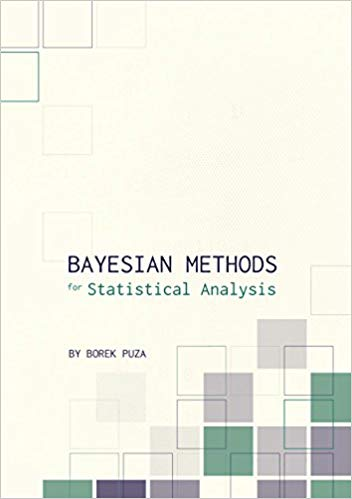Book Description

Bayesian methods for statistical analysis is a book on statistical methods for analysing a wide variety of data. The book consists of 12 chapters, starting with basic concepts and covering numerous topics, including Bayesian estimation, decision theory, prediction, hypothesis testing, hierarchical models, Markov chain Monte Carlo methods, finite population inference, biased sampling and nonignorable nonresponse.

The book contains many exercises, all with worked solutions, including complete computer code. It is suitable for self-study or a semester-long course, with three hours of lectures and one tutorial per week for 13 weeks.

• Dr Borek Puza teaches Statistics in the Research School of Finance, Actuarial Studies and Statistics. He has a BSc in Mathematics and a Graduate Diploma, Masters and PhD in Statistics.
Reviews, Ratings, and Recommendations: Related Book Categories: Read and Download Links:Similar Books:
•Bayes Rules! An Introduction to Applied Bayesian Modeling

An engaging, sophisticated, and fun introduction to the field of Bayesian statistics, it brings the power of modern Bayesian thinking, modeling, and computing to a broad audience. Integrates R code, including RStan modeling tools, bayesrules package.

•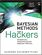Bayesian Methods for Hackers: Probabilistic Programming

This book illuminates Bayesian inference through probabilistic programming with the powerful PyMC language and the closely related Python tools NumPy, SciPy, Matplotlib, through practical examples and computation - no advanced mathematics required.

•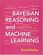Bayesian Reasoning and Machine Learning (David Barber)

This practical introduction is ideally suited to computer scientists without a background in calculus and linear algebra. You'll develop analytical and problem-solving skills that equip them for the real world. Numerous examples and exercises are provided.

•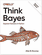O'Reilly® Think Bayes: Bayesian Statistics in Python

If you know how to program, you're ready to tackle Bayesian statistics. With this book, you'll learn how to solve statistical problems with Python code instead of mathematical formulas, using discrete probability distributions rather than continuous mathematics.

•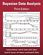Bayesian Data Analysis (Andrew Gelman, et al.)

This classic book is widely considered the leading text on Bayesian methods, lauded for its accessible, practical approach to analyzing data and solving research problems. It takes an applied approach to analysis using up-to-date Bayesian methods.

•An Introduction to Bayesian Thinking (Merlise Clyde, et al.)

This book provides an introduction to Bayesian inference in decision making without requiring calculus. It may be used on its own as an open-access introduction to Bayesian inference using R for anyone interested in learning about Bayesian statistics.

•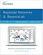Bayesian Networks and BayesiaLab (Stefan Conrady, et al.)

This practical introduction is geared towards scientists who wish to employ Bayesian Networks for applied research using the BayesiaLab software platform. It can serve as a self-study guide for learners and as a reference manual for advanced practitioners.

Book Categories
 :All CategoriesTop Free BooksRecent BooksMiscellaneous BooksComputer EngineeringComputer LanguagesComputer ScienceData Science/DatabasesJava and Java EE (J2EE)Linux and UnixMathematicsMicrosoft and .NETMobile ComputingNetworking and CommunicationsSoftware EngineeringSpecial TopicsWeb Programming
Other Categories